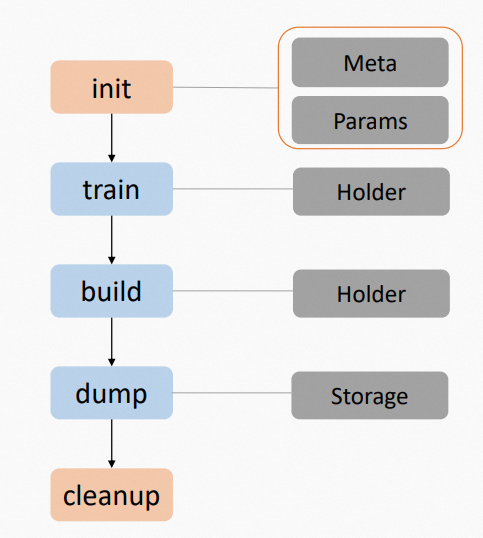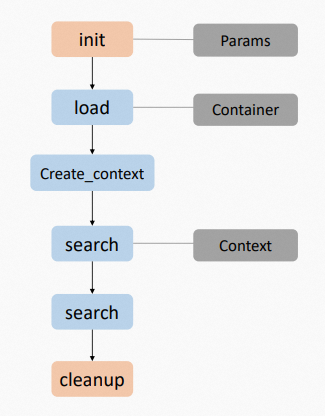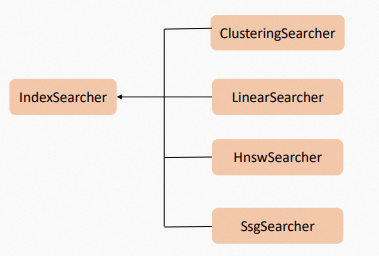## IndexBuilder

IndexBuilder为索引构建模块，其基本调用流程如下：1. 初始化Builder。
2. 数据训练。
3. 构建索引。
4. dump索引。
5. 清理资源。

## IndexConverter

IndexConverter是对特征向量进行转换的模块，例如对特征进行降维，Half FLOAT转换，INT8量化等。可独立使用，也可作为检索流程中一部分。

IndexConverter在检索流程中使用时，一般与IndexReformer结合使用，类似IndexBuilder与IndexSearcher的关系。Index Converter作为Index Builder流程前置环节，特征向量经过IndexConverter后，再进行索引的构建。在线检索时，所有Query向量先经过IndexReformer转换，再交由IndexSearcher进行检索。

## IndexMeasure

IndexMeasure为相似度（距离）计算模块，用于计算适配，遵从距离越小，相似度越近的原则。相关的IndexMeasure插件名及参数详情请参见IndexMeasure参数配置

### 距离计算公式

• 数值距离
距离参数计算公式
Squared Euclidean$$\sum_{i=0}^n (u_i - v_i)^2$$
Euclidean$$\sqrt{\sum_{i=0}^n (u_i - v_i)^2}$$
Normalized Euclidean$$\sqrt{\frac{1}{2}\frac{\sum_{i=0}^n [(u_i-\bar{u}) - (v_i-\bar{v})]^2}{\sum_{i=0}^n [(u_i-\bar{u})^2 + (v_i-\bar{v})^2]}}$$
Normalized Squared Euclidean$$\frac{1}{2}\frac{\sum_{i=0}^n [(u_i-\bar{u}) - (v_i-\bar{v})]^2}{\sum_{i=0}^n [(u_i-\bar{u})^2 + (v_i-\bar{v})^2]}$$
Manhattan$$\sum_{i=0}^n |u_i - v_i|$$
Chebyshev (Chessboard)$$\max_{i=0} |u_i - v_i|$$
Cosine$$1.0 - \frac{\sum_{i=0}^n u_iv_i}{\sqrt{\sum_{i=0}^n u_i^2}\sqrt{\sum_{i=0}^n v_i^2}}$$
Minus Inner Product$$-\sum_{i=0}^n u_iv_i$$
Canberra$$\sum_{i=0}^n\frac{|u_i-v_i|}{|u_i|+|v_i|}$$
Bray Curtis$$\frac{\sum_{i=0}^n|u_i-v_i|}{\sum_{i=0}^n|u_i+v_i|}$$
Correlation$$1.0 - \frac{\sum_{i=0}^n(u_i-\bar{u})(v_i-\bar{v})}{\sqrt{\sum_{i=0}^n(u_i-\bar{u})^2} \sqrt{\sum_{i=0}^n(v_i-\bar{v})^2}}$$
Binary$$[!u == v]$$
• 二值距离
距离参数计算公式
Hamming$$M_{10}+M_{01}$$
Jaccard$$\frac{M_{10}+M_{01}}{M_{11}+M_{10}+M_{01}}$$
Matching$$\frac{M_{10}+M_{01}}{M_{11}+M_{10}+M_{01}+M_{00}}=\frac{M_{10}+M_{01}}{N}$$
Dice$$\frac{M_{10}+M_{01}}{2M_{11}+M_{10}+M_{01}}$$
Rogers Tanimoto$$\frac{2(M_{10}+M_{01})}{M_{11}+2(M_{10}+M_{01})+M_{00}}$$
Russell Rao$$\frac{M_{10}+M_{01}+M_{00}}{N}$$
Sokal Michener$$\frac{M_{10}+M_{01}}{M_{11}+M_{10}+M_{01}+M_{00}}=\frac{M_{10}+M_{01}}{N}$$
Sokal Sneath I$$1.0 - \frac{M_{11}}{M_{11} + 2(M_{10}+M_{01})}=\frac{2(M_{10}+M_{01})}{M_{11}+2(M_{10}+M_{01})}$$
Sokal Sneath II$$1.0 - \frac{2(M_{11} + M_{00})}{2(M_{11} + M_{00}) + M_{10} + M_{01}} = \frac{M_{10} + M_{01}}{2N - (M_{10} + M_{01})}$$
Sokal Sneath III$$1.0 - \frac{M_{11} + M_{00}}{M_{10} + M_{01}} = \frac{2(M_{10} + M_{01}) - N}{M_{10} + M_{01}}$$
Sokal Sneath IV$$1.0 - \frac{1}{4}(\frac{M_{11}}{M_{11} + M_{10}} + \frac{M_{11}}{M_{11} + M_{01}} + \frac{M_{00}}{M_{10} + M_{00}} + \frac{M_{00}}{M_{01} + M_{00}})$$
Sokal Sneath V$$1.0 - \frac{M_{11}M_{00}}{\sqrt{(M_{11} + M_{10}) (M_{11} + M_{01}) (M_{10} + M_{00}) (M_{01} + M_{00})}}$$
Kulczynski I$$1.0-\frac{S_{AB}}{S_A+S_B-2S_{AB}} = 1.0-\frac{M_{11}}{M_{10}+M_{01}} = \frac{M_{10}+M_{01}-M_{11}}{M_{10}+M_{01}}$$
Kulczynski II$$1.0-\frac{1}{2}\left(\frac{S_{AB}}{S_{A}}+\frac{S_{AB}}{S_{B}}\right)$$
Yule$$\frac{2M_{10}M_{01}}{M_{11}M_{00}+M_{10}M_{01}}$$

## IndexSearcher

IndexSearcher是进行KNN检索的主要模块，以只读方式加载离线构建好的索引并进行在线检索。

IndexSearcher的调用流程如下：1. 初始化Searcher。
2. 加载索引数据。
3. 创建检索上下文 - 检索。
4. 执行查询。
5. 卸载索引数据。
6. 清理资源。
IndexSearcher支持并发检索，但因为使用方的场景和环境差异较大，所以需要将并发的控制权交给引擎使用者。为此，Proxima CE引进了检索上下文的概念，即Searcher Context，其保存了检索结果以及检索过程中的中间数据。每一个上下文（Context）对象可复用，但仅允许串行进入，不允许多个线程同时进入。实现并发检索时，需要创建多个上下文（Context）对象。目前Proxima内置了多种Searcher插件，如：ClusteringSearcher、LinearSearcher、HnswSearcher和SsgSearcher等。## IndexBuilder参数配置

• ClusteringBuilder
重要 proxima.hc.builder.max_document_countproxima.hc.builder.centroid_count至少设置其中一项。
参数名类型默认值说明
proxima.hc.builder.max_document_countUNIT32proxima.hc.builder.centroid_count未设置时，使用proxima.hc.builder.max_document_count计算中心点数量。
proxima.hc.builder.centroid_countSTRING聚类中心点参数，支持层次聚类。层之间用*分隔。该参数不指定会根据proxima.hc.builder.max_document_count自动推导。
• 一层聚类示例：1000
• 两层示例：100*100
如果使用两层中心点，一般第一层中心点数量比第二层多，效果更好。第一层经验值是第二层10倍。
• HnswBuilder
参数名类型默认值说明
proxima.hnsw.builder.efconstructionUNIT32500用于控制图的构建精度。该值越大，构建的图越精确，但构建更耗时。
proxima.hnsw.builder.max_neighbor_countUNIT32100图的邻居数。值越大，图越精确，但计算和存储开销越大，一般不超过特征维度。最大为65535个。
• SsgBuilder
参数名类型默认值说明
proxima.ssg.builder.efconstructionUNIT32500用于控制图的构建精度。该值越大，构建的图越精确，但构建更耗时。
proxima.ssg.builder.max_neighbor_countUNIT32100图的邻居数。值越大，图越精确，但计算和存储开销越大，一般不超过特征维度。最大为65535。
proxima.ssg.builder.centroid_countUNIT320训练样本产出的聚类中心点数量。中心点越多，构建成本越高，图精度也会越高。一般建议：
• 文档数<200W，中心点=2000
• 文档数200W~1KW，中心点=5000
• 文档数>1KW，中心点=8000
proxima.ssg.builder.scan_ratioFLOAT0.01聚类扫描数，默认是1%。该值控制图的精度，调整越高，图精度越高，但构图成本线性增加。一般建议根据文档数具体配置：
• 文档数<200W，ratio = 1W/doc_count
• 文档数200W~1KW， ratio = 2W/doc_count
• 文档数>1KW，ratio = 5W/doc_count
• GcBuilder
重要 proxima.gc.builder.centroid_count参数必须指定。
参数名类型默认值说明
proxima.gc.builder.centroid_countSTRING聚类中心点参数，支持层次聚类。层之间用*分隔。
• 一层聚类示例：1000
• 两层示例：100*100
如果使用两层中心点，一般第一层中心点数量比第二层多，效果更好。第一层经验值是第二层10倍。
• LinearBuilder
参数名类型默认值说明
proxima.linear.builder.column_major_orderSTRINGfalse构建的时候特征用行排（false）/列排（true）。
• QcBuilder
说明 proxima.qc.builder.centroid_count 参数必须指定。
参数名类型默认值说明
proxima.qc.builder.centroid_countSTRING聚类中心点参数，支持层次聚类。层之间用*分隔。
• 一层聚类示例：1000
• 两层示例：100*100
如果使用两层中心点，一般第一次中心点数量比第二层多，效果更好。经验值是第一层是第二层10倍。
proxima.qc.builder.quantizer_classSTRING配置量化器，默认不使用量化器。可选值有 Int8QuantizerConverter、HalfFloatConverter、DoubleBitConverter。一般配置量化器可提升性能，减少索引大小，召回视情况有所损失。
proxima.qc.builder.quantizer_paramsIndexParams配置上面量化器相关参数。

## IndexSearcher参数配置

• ClusteringSearcher
参数名类型默认值说明
proxima.hc.searcher.max_scan_countUNIT32在线查找文档截断数，用于控制考察范围。值越大一般召回率越多，但最多不会超过proxima.hc.searcher.scan_count_in level中指定的中心点下doc数量。
proxima.hc.searcher.scan_ratioFLOAT0.01用于计算max_scan_count数量，总doc数量 * scan_ratio
• HnswSearcher
参数名类型默认值说明
proxima.hnsw.searcher.efUNIT32500在线查找文档截断数，用于控制考察范围。值越大一般召回率越多，但最多不会超过proxima.hc.searcher.scan_count_in level中指定的中心点下doc数量。
proxima.hnsw.searcher.max_scan_ratioFLOAT0.1f用于计算max_scan_count数量，总doc数量 * scan_ratio
proxima.hnsw.searcher.brute_force_thresholdINT1000当总doc数量小于此值时，走线性检索。
• SsgSearcher
参数名类型默认值说明
proxima.ssg.searcher.efUNIT32500用于检索时，考察精度。该值越大，扫描doc数越多，召回率越高。
proxima.ssg.searcher.max_scan_ratioUNIT320最大扫描文档比例，兜底截断策略，默认0代表不开启。
• GcSearcher
参数名类型默认值说明
proxima.gc.searcher.scan_ratioFLOAT0.01用于计算max_scan_count数量，总doc数量 * scan_ratio
• LinearSearcher
参数名类型默认值说明
• QcSearcher
参数名类型默认值说明
proxima.qc.searcher.scan_ratioFLOAT0.01用于计算max_scan_count数量，总doc数量 * scan_ratio
proxima.qc.searcher.brute_force_thresholdINT1000如果总doc数小于此值，则走线性检索。

## IndexConverter参数配置

• MipsConverter
参数名类型默认值说明
proxima.mips.converter.m_valueUINT32M值，即增加的维数，一般不超过4。
proxima.mips.converter.u_valueFLOAT0.38196601U值，取值范围：0~1.0。
proxima.mips.converter.forced_half_floatBOOLEANfalse强制将FP32的数据转换为FP16。
proxima.mips.converter.spherical_injectionBOOLEANfalse是否采用spherical injection的变换方式，只增加1维。
• HalfFloatConverter

无需进行参数配置。

• DoubleBitConverter
参数名类型默认值说明
proxima.double_bit.converter.train_sample_countINT0进行训练的数据量，如果为0，则使用holder全量数据。
• Int8QuantizerConverter

无需进行参数配置。

• Int4QuantizerConverter

无需进行参数配置。

• NormalizeConverter
参数名类型默认值说明
proxima.normalize.reformer.forced_half_floatBOOLEANfalse强制将FP32的数据转换为FP16。
proxima.normalize.reformer.p_valueUNIT322使用P-norm中的P值。

## IndexReformer参数配置

• MipsReformer
参数名类型默认值说明
proxima.mips.reformer.m_valueUINT324M值，即增加的维数，一般不超过 4。
proxima.mips.reformer.u_valueFLOAT0.38196601U值，取值范围：大于 0，小于 1.0。
proxima.mips.reformer.l2_normFLOAT0.0训练得到的L2 NORM值。
proxima.mips.reformer.normalizeBOOLEANfalse是否归一化。
proxima.mips.reformer.forced_half_floatBOOLEANfalse强制将FP32的数据转换为FP16。
• HalfFloatReformer

无需进行参数配置。

## IndexMeasure参数配置

• Canberra

无需进行参数配置。

• Chebyshev

无需进行参数配置。

• SquaredEuclidean

无需进行参数配置。

• Euclidean

无需进行参数配置。

• GeographicalDistance

无需进行参数配置。

• Hamming

无需进行参数配置。

• InnerProduct

无需进行参数配置。

• Manhattan

无需进行参数配置。

• Matching

无需进行参数配置。

• MipsSquaredEuclidean
参数名类型默认值说明
proxima.mips_euclidean.measure.injection_typeINT0对内积特征变换注入方式：
• 0 LocalizedSpherical
• 1 Spherical